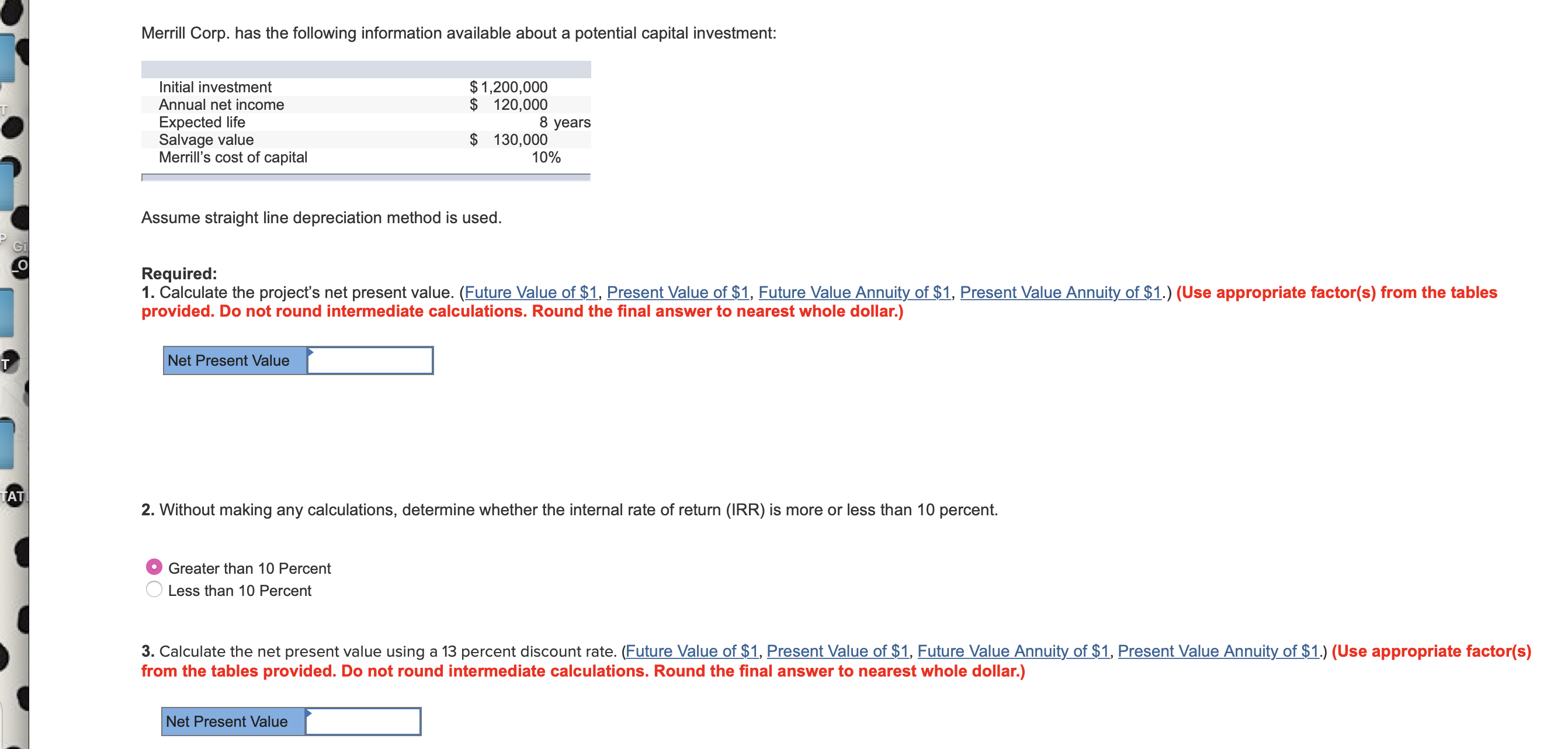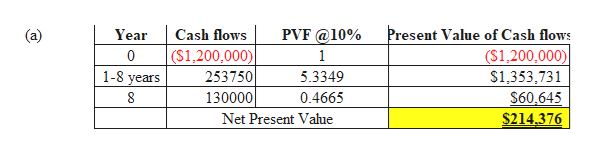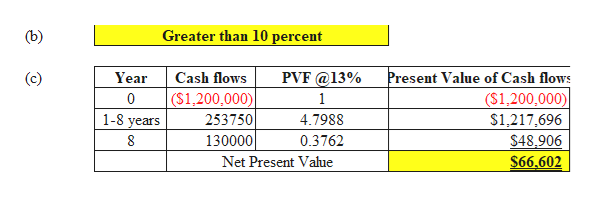# Merrill Corp. has the following information available about a potential capital investment:\$1,200,000\$ 120,0008 yearsInitial investmentAnnual net incomeTExpected lifeSalvage valueMerrill's cost of capital130,00010%Assume straight line depreciation method is used.GiоRequired:1. Calculate the project's net present value. (Future Value of \$1, Present Value of \$1, Future Value Annuity of \$1, Present Value Annuity of \$1.) (Use appropriate factor(s) from the tablesprovided. Do not round intermediate calculations. Round the final answer to nearest whole dollar.)Net Present ValueTATRR) is mor less than 10 percent.2. Without making any calculations, determine whether the internal rate of returnGreater than 10 PercentLess than 10 Percent3. Calculate the net present value using a 13 percent discount rate. (Future Value of \$1, Present Value of \$1, Future Value Annuity of \$1, Present Value Annuity of \$1.) (Use appropriate factor(s)from the tables provided. Do not round intermediate calculations. Round the final answer to nearest whole dollar.)Net Present Value

Question
40 views

how would i solve this problemhelp_outlineImage TranscriptioncloseMerrill Corp. has the following information available about a potential capital investment: \$1,200,000 \$ 120,000 8 years Initial investment Annual net income T Expected life Salvage value Merrill's cost of capital 130,000 10% Assume straight line depreciation method is used. Gi о Required: 1. Calculate the project's net present value. (Future Value of \$1, Present Value of \$1, Future Value Annuity of \$1, Present Value Annuity of \$1.) (Use appropriate factor(s) from the tables provided. Do not round intermediate calculations. Round the final answer to nearest whole dollar.) Net Present Value TAT RR) is m or less than 10 percent. 2. Without making any calculations, determine whether the internal rate of return Greater than 10 Percent Less than 10 Percent 3. Calculate the net present value using a 13 percent discount rate. (Future Value of \$1, Present Value of \$1, Future Value Annuity of \$1, Present Value Annuity of \$1.) (Use appropriate factor(s) from the tables provided. Do not round intermediate calculations. Round the final answer to nearest whole dollar.) Net Present Value fullscreen
check_circle

Step 1help_outlineImage TranscriptionclosePresent Value of Cash flows PVF @10% Cash flows Year (\$1,200,000) (\$1,200,000) 0 1 1-8 years 253750 5.3349 S1,353,731 0.4665 8 130000 S60,645 Net Present Vahue \$214.376 fullscreen
Step 2help_outlineImage TranscriptioncloseGreater than 10 percent (b) Present Value of Cash flows (\$1,200,000) PVF@13% (c) Year Cash flows (\$1,200,000) 1 1-8 years 253750 4.7988 \$1,217,696 8 130000 0.3762 \$48,906 \$66.602 Net Present Value fullscreen

### Want to see the full answer?

See Solution

#### Want to see this answer and more?

Solutions are written by subject experts who are available 24/7. Questions are typically answered within 1 hour.*

See Solution
*Response times may vary by subject and question.
Tagged in